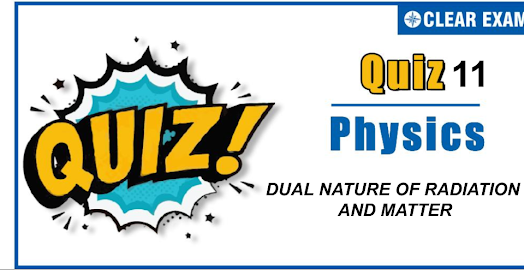## DUAL NATURE OF RADIATION AND MATTER QUIZ-11

As per analysis for previous years, it has been observed that students preparing for NEET find Physics out of all the sections to be complex to handle and the majority of them are not able to comprehend the reason behind it. This problem arises especially because these aspirants appearing for the examination are more inclined to have a keen interest in Biology due to their medical background. Furthermore, sections such as Physics are dominantly based on theories, laws, numerical in comparison to a section of Biology which is more of fact-based, life sciences, and includes substantial explanations. By using the table given below, you easily and directly access to the topics and respective links of MCQs. Moreover, to make learning smooth and efficient, all the questions come with their supportive solutions to make utilization of time even more productive. Students will be covered for all their studies as the topics are available from basics to even the most advanced. .

Q1. In a discharge tube at 0.02 mm, there is a formation of
•  FDS
•  CDS
•  Both space
•  None of these
Solution
CDS

Q2.Monochromatic radiation emitted when electron on hydrogen atom jumps from first excited to the ground state irradiates a photosensitive material. The stopping potential is measured to be 3.57 V. the threshold frequency of the material is
•  4×1015 Hz
•  5×1015 Hz
•  1.6×1015 Hz
•  2.5×1015 Hz
Solution
n→2-1 E=10.2 eV kE=E-ϕ Q=10.20-3.57 hv0=6.63 eV v0=(6.63×1.6×10-19)/(6.67×10-34 )=1.6×1015

Q3. The curve between current (i)and potential difference(V) for a photo cell will be
Solution
In photocell, at a particular negative potential (stopping potential V0) of anode, photoelectric current is zero, as the potential difference between cathode and anode increases current through the circuit increases but after some time constant current (saturation current) flows through the circuit even if potential difference still increases

Q4. Penetrating power of X-rays can be increased by
•  Increasing the potential difference between anode and cathode
•  Decreasing the potential difference between anode and cathode
•  Increasing the cathode filament current
•  Decreasing the cathode filament current
Solution
With the increase in potential difference between anode and cathode energy of striking electrons increases which in turn increses the energy (penetration power) of X-rays

Q5.In an experiment on photoelectric emission from a metallic surface, wavelength of incident light is 2×10-7 and stopping potential is 2.5 V. The threshold frequency of the metal (in Hz ) approximately (charge on electron e=1.6×10-19 C, Planck’s constant h=6.6×10-34J-s)
•  12×1015
•  9×1015
•  9×1014
•  12×1013
Solution
eV0=hv-hv0 ∴ Threshold frequency, v0=v-(eV0)/h = c/λ-(eV0)/h ∴ v0=(3×108)/(2×10-7 )-(1.6×10-19×2.5)/(6.6×10-34 ) = 9.0×1014 Hz

Q6. The maximum wavelength of radiation that can produce photoelectric effect in certain metal is 200 nm. The maximum kinetic energy acquired by electron due to radiation of wavelength 100 nm will be
•  12.4 eV
•  6.2 eV
• 100 eV
•  200 eV
Solution
Here, λ0=200nm; λ=100nm; hc/e = 1240eV nm maximum KE =hc/λe-hc/(λ0 e)(in eV) =hc/e (1/λ-1/λ0 ) =1240(1/100-1/200) =6.2 eV

Q7.From the following, what charges can be present on oil drops in Millikan’s experiment (Here e is the electronic charge)
•  Zero, equal to the magnitude of charge on α-particle
•  2e,1.6×10-18 C
•  1.6×10-19 C,2.5e
•  1.5e,e
Solution
In Milikan’s experiment, the charges present on the oil drops are the integral multiples of electronic charge, so 2e and 10e(1.6×10-18 C) charges are present

Q8.Which of the following metal thermionically emits an electron at a relatively lowest temperature among them
•  Platinum
•  TCopper
•  Aluminium
•  Molybdenum
Solution
Among the given metals, aluminium thermionically emits an electron at a relatively lowest temperature

Q9.The temperature at which protonsin proton gas would have enough energy to overcome Coulomb barrier of 4.14×10-14 J is (Boltzmann constant =1.38×10-23 JK-1)
•  2×109 K
•  109 K
•  6×109 K
•  3×109 K
Solution
Given k=1.38×10-23 JK-1 The energy of proton gas =4.14×10-14 J E=3/2 kT 4.14×10-14=3/2×1.38×10-23×T T=2×109 K.

Q10. The longest wavelength that can be analysed by a sodium chloride crystal of spacing d=2.82 A° in the second order is
•  2.82 A°
•  5.64 A°
•  8.46 A°
• 11.28 A°
Solution
Use Bragg’s X-ray diffraction Law nλ=2d sin⁡θ ∴λ=(2d sin⁡θ)/n For longest wavelength take sin θ=1 ∴λ=(2×2.82[Å])/2[∵n=2 for second order] =2.82 Å## Want to know more

Please fill in the details below:

## Latest NEET Articles\$type=three\$c=3\$author=hide\$comment=hide\$rm=hide\$date=hide\$snippet=hide

Name

ltr
item
BEST NEET COACHING CENTER | BEST IIT JEE COACHING INSTITUTE | BEST NEET, IIT JEE COACHING INSTITUTE: QUIZ-11 DUAL NATURE OF RADIATION AND MATTER
QUIZ-11 DUAL NATURE OF RADIATION AND MATTER
https://1.bp.blogspot.com/-4n2kPo0iAq4/X50y4WlLDOI/AAAAAAAACa4/1nArQ5VTdTAfeg_IOX7ZZLM2q61Y4e6RwCLcBGAsYHQ/w573-h275/Quiz%2BImage%2B20%2B%252812%2529.png
https://1.bp.blogspot.com/-4n2kPo0iAq4/X50y4WlLDOI/AAAAAAAACa4/1nArQ5VTdTAfeg_IOX7ZZLM2q61Y4e6RwCLcBGAsYHQ/s72-w573-c-h275/Quiz%2BImage%2B20%2B%252812%2529.png
BEST NEET COACHING CENTER | BEST IIT JEE COACHING INSTITUTE | BEST NEET, IIT JEE COACHING INSTITUTE